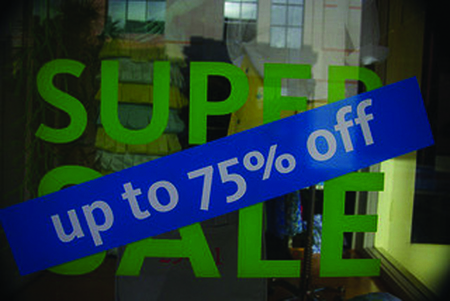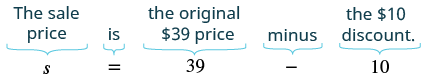## Solving Discount and Markup Applications

### Learning Outcomes

• Solve discount applications
• Solve mark-up applications

Applications of discount are very common in retail settings (see the image below). When you buy an item on sale, the original price of the item has been reduced by some dollar amount. The discount rate, usually given as a percent, is used to determine the amount of the discount. To determine the amount of discount, we multiply the discount rate by the original price. We summarize the discount model in the box below.Applications of discounts are common in everyday life. (credit: Charleston’s TheDigitel, Flickr)

### Discount

An amount of discount is a percent off the original price.

$\begin{array}{ccc}\hfill \text{amount of discount}& =& \text{discount rate}\cdot \text{original price}\hfill \\ \hfill \text{sale price}& =& \text{original price}-\text{discount}\hfill \end{array}$

The sale price should always be less than the original price. In some cases, the amount of discount is a fixed dollar amount. Then we just find the sale price by subtracting the amount of discount from the original price.

### example

Jason bought a pair of sunglasses that were on sale for $\text{\10}$ off. The original price of the sunglasses was $\text{\39}$. What was the sale price of the sunglasses?

Solution

 Identify what you are asked to find. What is the sale price? Choose a variable to represent it. Let $s=$ the sale price. Write a sentence that gives the information to find it. The sale price is the original price minus the discount. Translate into an equation.Simplify. $s=29$ Check if this answer is reasonable. Yes. The sale price, $\text{\29}$, is less than the original price, $\text{\39}$. Write a complete sentence that answers the question. The sale price of the sunglasses was $\text{\29}$.

### try it

In the first example, the amount of discount was a set amount, $\text{\10}$. In the next example, the discount is given as a percent of the original price.

### exmaple

Elise bought a dress that was discounted $\text{35%}$ off of the original price of $\text{\140}$. What was ⓐ the amount of discount and ⓑ the sale price of the dress?

### Example

Jeff has a coupon at the Guitar Store for $15\%$ off any purchase of $\100$ or more. He wants to buy a used guitar that has a price tag of $\220$ on it. Jeff wonders how much money the coupon will take off of the  $\220$ original price.

You can estimate to see if the answer is reasonable. Since  $15\%$ is half way between  $10\%$ and $20\%$, find these numbers.

$\begin{array}{c}10\%\,\,\text{of}\,\,220=0.1\cdot220=22\\20\%\,\,\text{of}\,\,220=0.2\cdot220=44\end{array}$

The answer, $33$, is between $22$ and $44$. So $33$ seems reasonable.

The example video that follows shows how to use the percent equation to find the amount of a discount from the price of a phone.

### Calculate Discount Rate

There may be times when you buy something on sale and want to know the discount rate. The next example will show this case.

### example

Jeannette bought a swimsuit at a sale price of $\text{\13.95}$. The original price of the swimsuit was $\text{\31}$. Find ⓐ the amount of discount and ⓑ the discount rate.

### try it

In the following video we show another example of how to find the discount rate (also called the percent of change) given the original price and the marked-down price.

## Solve Mark-up Applications

Applications of mark-up are very common in retail settings. The price a retailer pays for an item is called the wholesale price. The retailer then adds a mark-up to the wholesale price to get the list price, the price he sells the item for. The mark-up is usually calculated as a percent of the wholesale price. The percent is called the mark-up rate. To determine the amount of mark-up, multiply the mark-up rate by the wholesale price. We summarize the mark-up model in the box below.

### Mark-up

The mark-up is the amount added to the wholesale price.

$\begin{array}{ccc}\hfill \text{amount of mark-up}& =& \text{mark-up rate}\cdot \text{wholesale price}\hfill \\ \hfill \text{list price}& =& \text{wholesale price}+\text{mark up}\hfill \end{array}$

The list price should always be more than the wholesale price.

### example

Adam’s art gallery bought a photograph at the wholesale price of $\text{\250}$. Adam marked the price up $\text{40%}$. Find ⓐ the amount of mark-up and ⓑ the list price of the photograph.

## Contribute!

Did you have an idea for improving this content? We’d love your input.